Grade B Standard Form Understand use standard form

• Slides: 19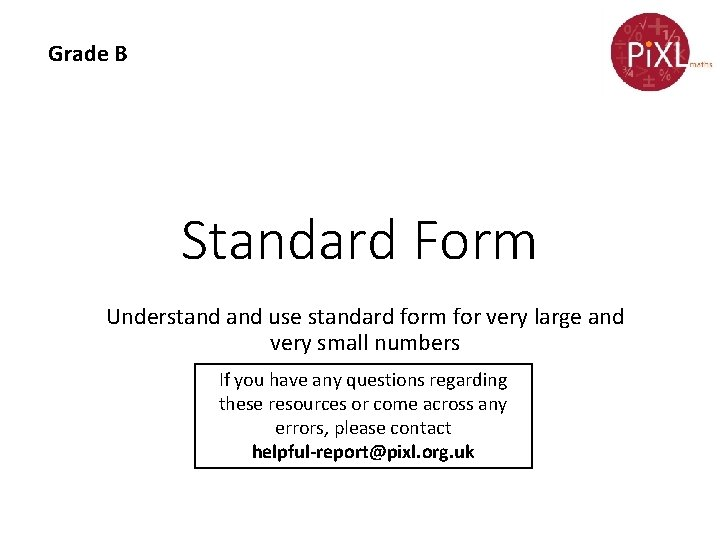Grade B Standard Form Understand use standard form for very large and very small numbers If you have any questions regarding these resources or come across any errors, please contact [email protected] org. ukKey Vocabulary Power Index notation Standard index form Scientific notation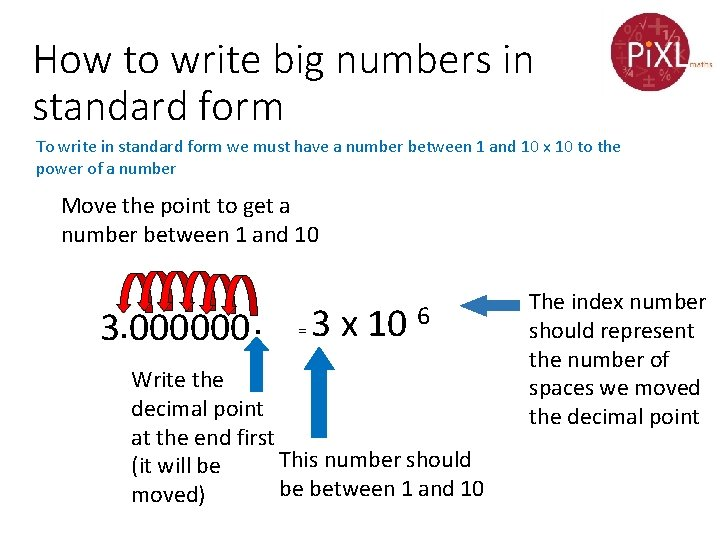How to write big numbers in standard form To write in standard form we must have a number between 1 and 10 x 10 to the power of a number Move the point to get a number between 1 and 10 3. 000000. = 3 x 10 6 Write the decimal point at the end first This number should (it will be be between 1 and 10 moved) The index number should represent the number of spaces we moved the decimal point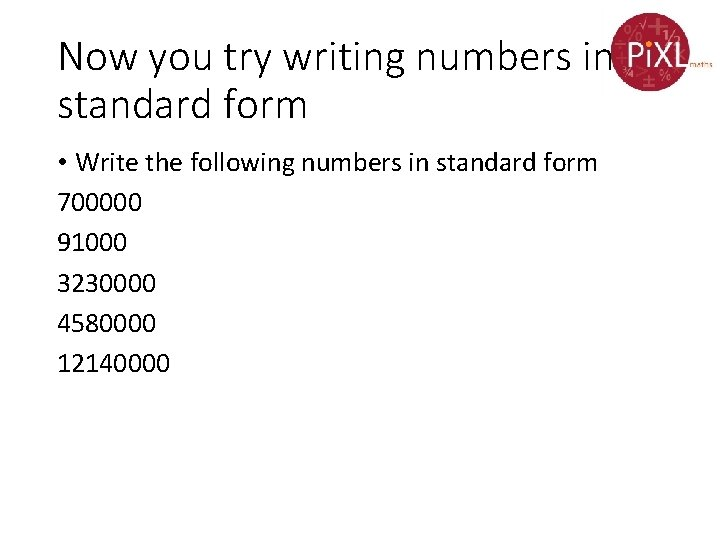Now you try writing numbers in standard form • Write the following numbers in standard form 700000 91000 3230000 4580000 12140000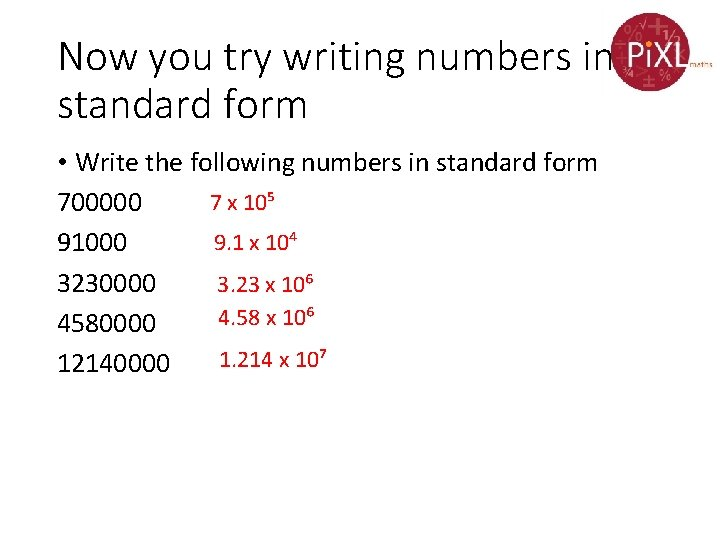Now you try writing numbers in standard form • Write the following numbers in standard form 7 x 10⁵ 700000 9. 1 x 10⁴ 91000 3230000 3. 23 x 10⁶ 4. 58 x 10⁶ 4580000 1. 214 x 10⁷ 12140000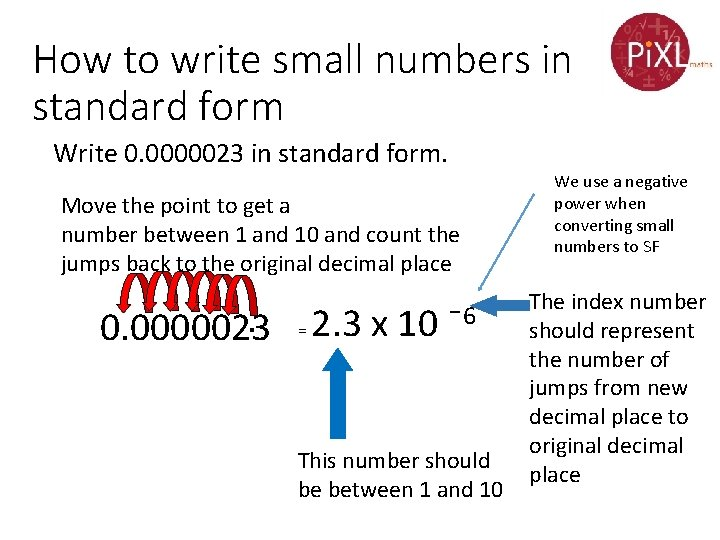How to write small numbers in standard form Write 0. 0000023 in standard form. Move the point to get a number between 1 and 10 and count the jumps back to the original decimal place . = 2. 3 x 10 ¯ 6 0. 0000023 This number should be between 1 and 10 We use a negative power when converting small numbers to SF The index number should represent the number of jumps from new decimal place to original decimal placeNow you try writing small numbers in standard form 0. 000035 0. 0025 0. 00000006 0. 00000789 0. 0000124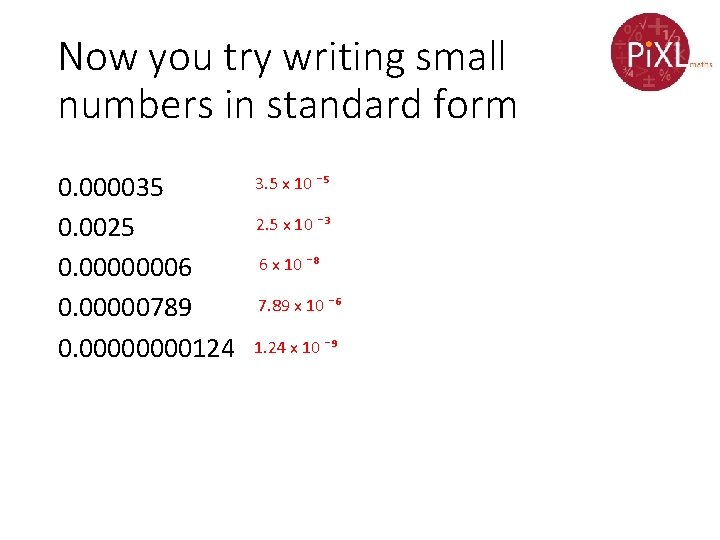Now you try writing small numbers in standard form 0. 000035 0. 0025 0. 00000006 0. 00000789 0. 0000124 3. 5 x 10 ¯⁵ 2. 5 x 10 ¯³ 6 x 10 ¯⁸ 7. 89 x 10 ¯⁶ 1. 24 x 10 ¯⁹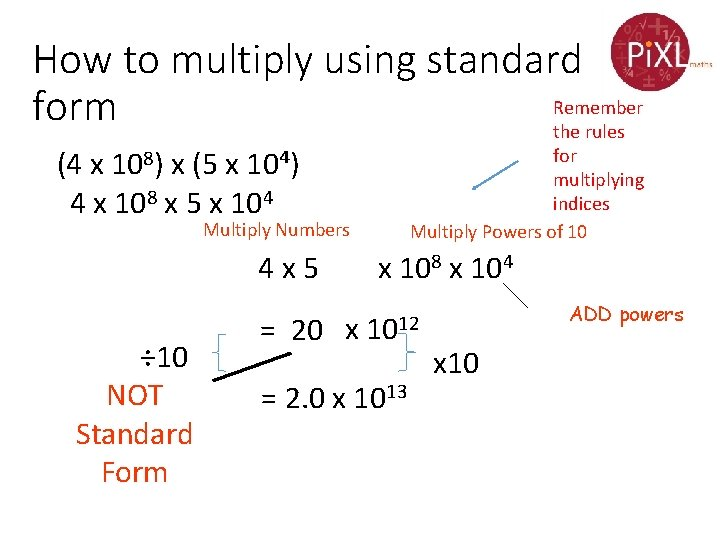How to multiply using standard Remember form the rules for multiplying indices Multiply Powers of 10 (4 x 108) x (5 x 10⁴) 4 x 108 x 5 x 104 Multiply Numbers 4 x 5 ÷ 10 NOT Standard Form = 20 x x 108 x 104 1012 = 2. 0 x 1013 ADD powers x 10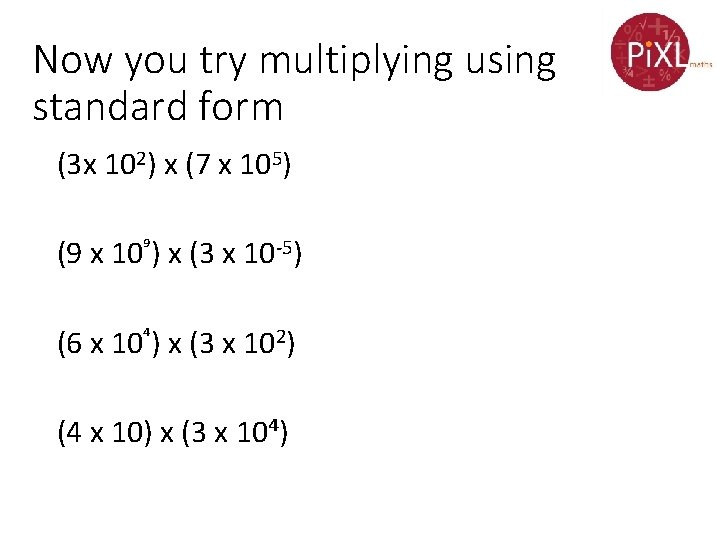Now you try multiplying using standard form (3 x 102) x (7 x 105) (9 x 10⁹) x (3 x 10 -5) (6 x 10⁴) x (3 x 102) (4 x 10) x (3 x 10⁴)Now you try multiplying using standard form (3 x 102) x (7 x 105) 2. 1 x 10⁸ (9 x 10⁹) x (3 x 10 -5) 2. 7 x 10⁵ (6 x 10⁴) x (3 x 102) 1. 8 x 10⁷ (4 x 10) x (3 x 10⁴) 1. 2 x 10⁵How to divide using standard form (44 x 108) ÷ (4 x 10⁴) Divide numbers 44 ÷ 4 = 11 x NOT Standard Form ÷ 10 Divide Powers of 10 108 ÷ 104 10⁴ = 1. 1 x 10⁵ subtract powers x 10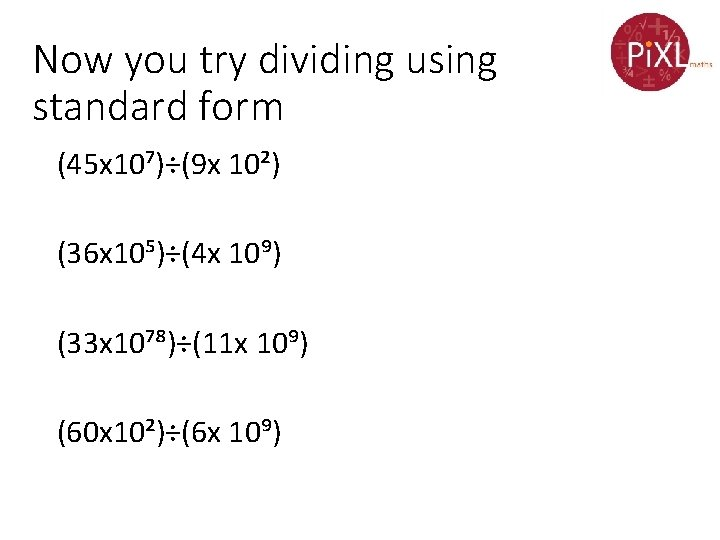Now you try dividing using standard form (45 x 10⁷)÷(9 x 10²) (36 x 10⁵)÷(4 x 10⁹) (33 x 10⁷⁸)÷(11 x 10⁹) (60 x 10²)÷(6 x 10⁹)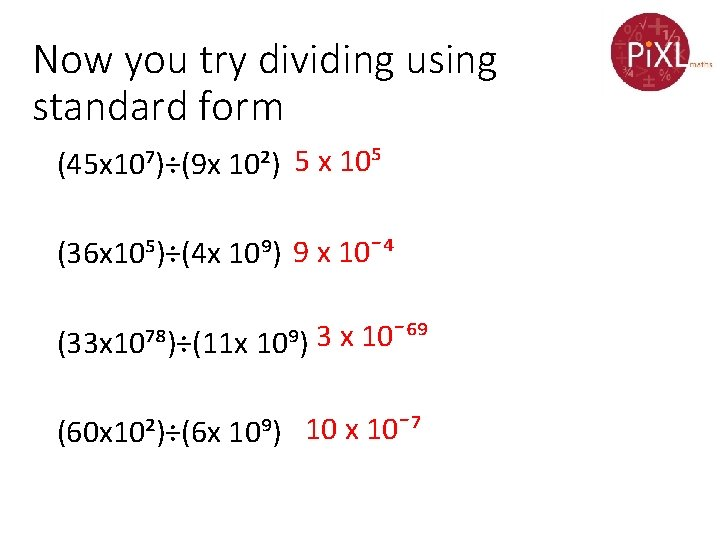Now you try dividing using standard form (45 x 10⁷)÷(9 x 10²) 5 x 10⁵ (36 x 10⁵)÷(4 x 10⁹) 9 x 10¯⁴ (33 x 10⁷⁸)÷(11 x 10⁹) 3 x 10¯⁶⁹ (60 x 10²)÷(6 x 10⁹) 10 x 10¯⁷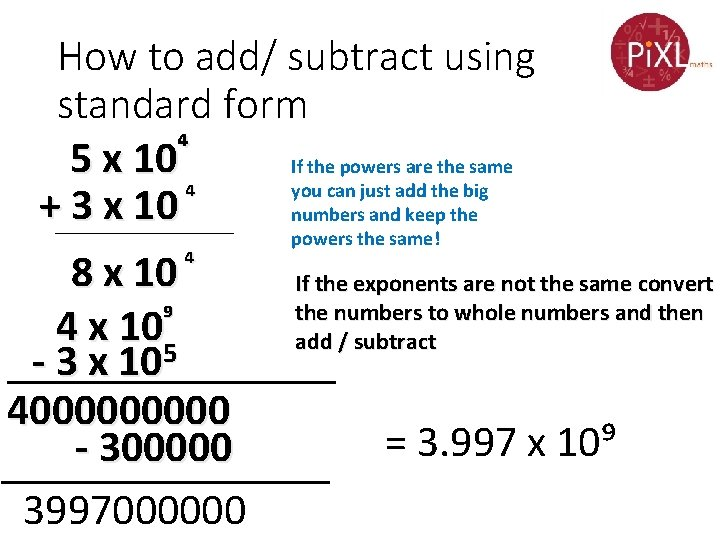How to add/ subtract using standard form 5 x 10⁴ If the powers are the same you can just add the big ⁴ +________ 3 x 10 numbers and keep the powers the same! 8 x 10 ⁴ If the exponents are not the same convert the numbers to whole numbers and then ⁹ 4 x 105 add / subtract - 3 x 10 ________ 400000 = 3. 997 x 10⁹ 300000 ________ 3997000000Now you try adding/ subtracting using standard form (2. 53 × 10 ⁹ ) + (7. 61 × 10⁸ ) (2. 86 × 10³ ) + (7. 55 × 10⁶ ) (2. 24 × 10² ) + (9. 92 × 10² ) (1. 35 × 10 ¯⁸ ) + (6. 82 × 10 ¯⁸ ) (1. 53 × 10¯³ ) - (2. 41 × 10¯⁴ ) (8. 22 × 10 ¯⁴ ) - (8. 33 × 10 ¯⁵ ) (2. 75 × 10 ¯⁴ ) - (4. 89 × 10 ¯⁷ )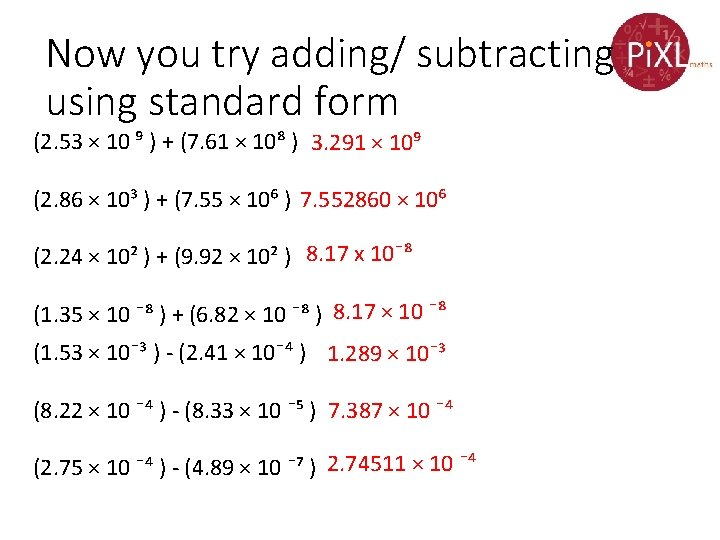Now you try adding/ subtracting using standard form (2. 53 × 10 ⁹ ) + (7. 61 × 10⁸ ) 3. 291 × 10⁹ (2. 86 × 10³ ) + (7. 55 × 10⁶ ) 7. 552860 × 10⁶ (2. 24 × 10² ) + (9. 92 × 10² ) 8. 17 x 10¯⁸ (1. 35 × 10 ¯⁸ ) + (6. 82 × 10 ¯⁸ ) 8. 17 × 10 ¯⁸ (1. 53 × 10¯³ ) - (2. 41 × 10¯⁴ ) 1. 289 × 10¯³ (8. 22 × 10 ¯⁴ ) - (8. 33 × 10 ¯⁵ ) 7. 387 × 10 ¯⁴ (2. 75 × 10 ¯⁴ ) - (4. 89 × 10 ¯⁷ ) 2. 74511 × 10 ¯⁴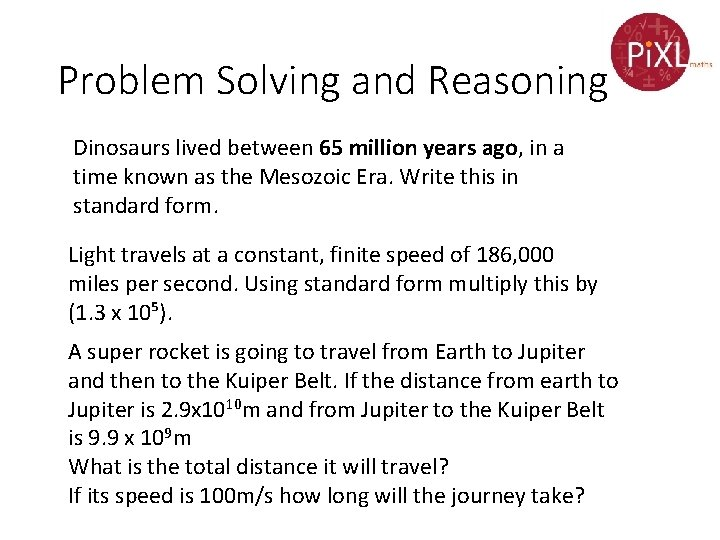Problem Solving and Reasoning Dinosaurs lived between 65 million years ago, in a time known as the Mesozoic Era. Write this in standard form. Light travels at a constant, finite speed of 186, 000 miles per second. Using standard form multiply this by (1. 3 x 10⁵). A super rocket is going to travel from Earth to Jupiter and then to the Kuiper Belt. If the distance from earth to Jupiter is 2. 9 x 1010 m and from Jupiter to the Kuiper Belt is 9. 9 x 109 m What is the total distance it will travel? If its speed is 100 m/s how long will the journey take?Problem Solving and Reasoning Dinosaurs lived between 65 million years ago, in a time known as the Mesozoic Era. Write this in standard form. 6. 5 x 10⁹ Light travels at a constant, finite speed of 186, 000 miles per second. Using standard form multiply this by (1. 3 x 10⁵). 2. 418 x 1010 A super rocket is going to travel from Earth to Jupiter and then to the Kuiper Belt. If the distance from earth to Jupiter is 2. 9 x 1010 m and from Jupiter to the Kuiper Belt is 9. 9 x 109 m What is the total distance it will travel? 3. 89 x 1010 If its speed is 100 m/s how long will the journey take? 3. 89 x 10⁸ seconds